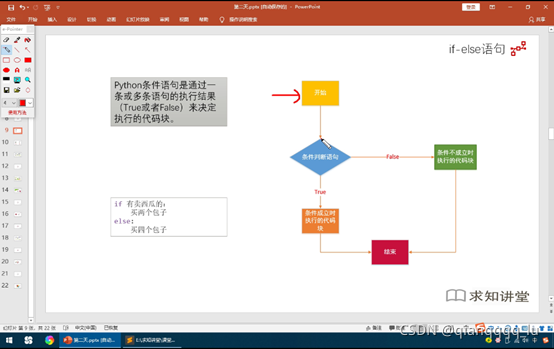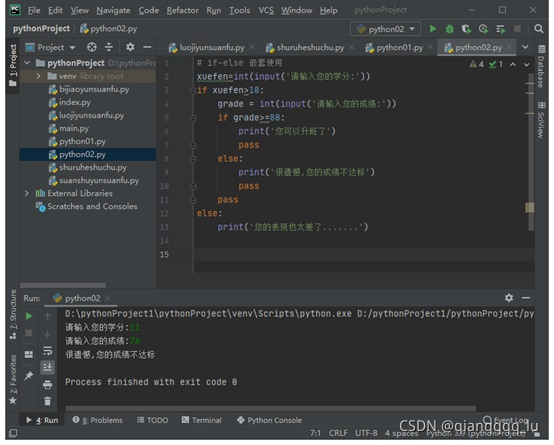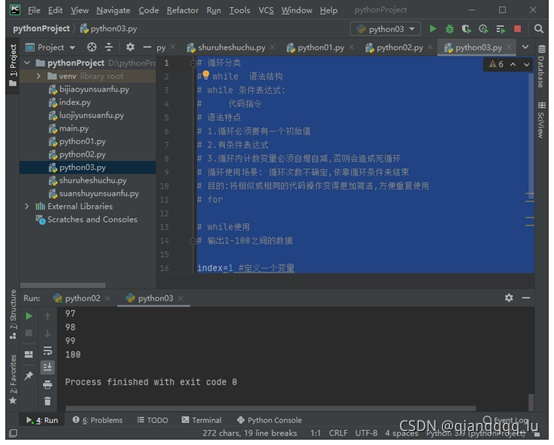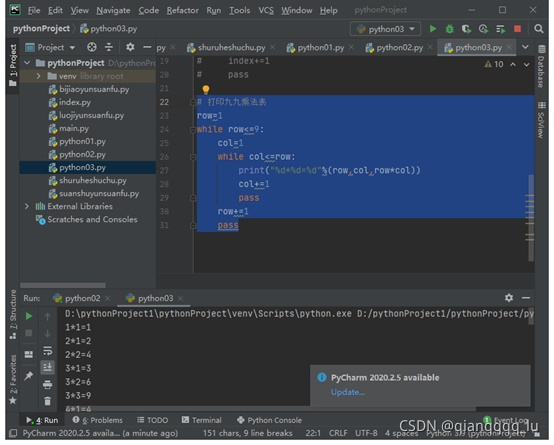﻿ python基础之循环语句_python_脚本之家
python# python基础之循环语句

## 循环语句## 单分支如何使用

```# 单分支表示
# if 条件表达式; 比较运算符/逻辑运算符 或者复合表达
#     代码指令
#     ......

score=60
if score<=60:  #满足条件就会输出打印提示
print('成绩不是太理想')
pass #空语句，结束跳过，用于填补结构
print('语句运行结束')
```## 双分支结构

```# 双分支
# if 条件表达式; 比较运算符/逻辑运算符 或者复合表达
#     代码指令
# else：
#     代码指令
#     ......
#  结果必定会执行其中一个分支

if score>60:
print('成绩合格')
pass
else:
print('成绩不合格')
pass
```## 多分支的使用

```# 多分支[多个条件]
# if 条件表达式; 比较运算符/逻辑运算符 或者复合表达
#     代码指令
# elif 条件表达式：
#     代码指令
# elif 条件表达式:
#     代码指令
# else:  ##实际情况可以没有
#     ......  ##特征必会满足其中一个
# 只要满足其中一个分支,就会退出本次if语句结构
# 至少存在两种以上情况可以选择
# elif之后必须跟上一个条件
# else是一个选配,根据实际情况来进行选择

score=int(input('请输入成绩:')) if score>90:
print('您的成绩为优秀')
pass elif score>80:
print('良好')
pass elif score>70:
print('中等')
pass elif score>=60:
print('合格')
pass else:
print('不合格')
pass
``````# 多分支多条件演练
# 猜拳击游戏
# 0石头 1剪刀 2布
import random  #导入随机数模块
# 计算机 人
person=int(input('请出拳:[0石头 1剪刀 2布]'))
computer=random.randint(0,2)
if person==0 and computer==1: #多条件
print('你赢啦....')
pass
elif person==1 and computer==2:
print('你赢啦....')
pass
elif person==2 and computer==0:
print('你赢啦....')
pass
elif person==computer:
print('不错,平手')
pass
else:
print('输啦......')
pass
print('程序执行完毕')
``````# if-else 嵌套使用
# 用在一个场景需要分阶段或者层次，做出不同的处理
# 要执行内部的条件 if 语句一定要外部的if语句 满足条件才可以
xuefen=int(input('请输入您的学分:'))
if xuefen>10:
print('您可以升班了')
pass
else:
print('很遗憾,您的成绩不达标')
pass
pass
else:
print('您的表现也太差了.......')
```## While 循环

```# 循环分类

#  while  语法结构
# while 条件表达式:
#     代码指令
# 语法特点
# 1.循环必须要有一个初始值
# 2.有条件表达式
# 3.循环内计数变量必须自增自减,否则会造成死循环
# 循环使用场景: 循环次数不确定,依靠循环条件来结束
# 目的:将相似或相同的代码操作变得更加简洁,方便重复使用
# for

# while使用
# 输出1-100之间的数据

index=1 #定义一个变量
while index<=100:
print(index)
index+=1  #变量的自增
pass
```## 拳击游戏循环：

```# 多分支多条件演练
# 猜拳击游戏
# 0石头 1剪刀 2布
import random  #导入随机数模块
# 计算机 人
count=1
while count<=10:
count+=1
person=int(input('请出拳:[0石头 1剪刀 2布]'))
computer=random.randint(0,2)
if person==0 and computer==1: #多条件
print('你赢啦....')
pass
elif person==1 and computer==2:
print('你赢啦....')
pass
elif person==2 and computer==0:
print('你赢啦....')
pass
elif person==computer:
print('不错,平手')
pass
else:
print('输啦......')
pass
print('程序执行完毕')
``````# 打印九九乘法表
row=1
while row<=9:
col=1
while col<=row:
print("%d*%d=%d"%(row,col,row*col))
col+=1
pass
row+=1
pass
``````# 打印九九乘法表
row=1
while row<=9:
col=1
while col<=row:
print("%d*%d=%d"%(row,col,row*col),end=" ")
col+=1
pass
print()
row+=1
pass
``````# 打印九九乘法表
row=9
while row>=1:
col=1
while col<=row:
print("%d*%d=%d"%(row,col,row*col),end=" ")
col+=1
pass
print()
row-=1
pass
``````# 打印直角三角形
row=1
while row<=7:
j=1
while j<=row:
print('*',end=' ')
j+=1
pass
print()
row+=1
pass
``````# 打印直角三角形
row=7
while row>=1:
j=1
while j<=row:
print('*',end=' ')
j+=1
pass
print()
row-=1
pass
``````# 打印等腰三角形
# 打印两类符号 空格和*
row=1
while row <= 5:
j=1
while j<=5-row: #控制打印空格
print(' ',end='')
j+=1
pass
k=1
while k<=2*row-1:   #控制打印*
print('*',end='')
k+=1
pass
print()
row+=1
```